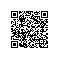# 当javaScript从入门到提高前需要注意的细节：变量部分

1. <script type="text/javascript">
2.
3.     var x = 10; //全局
4.     y = 100; //全局
5.
6.     function fun1() {
7.         var m = 99; //局部
8.         n = 9; //全局
9.     }
10.
15.     fun1();
18.
19.
20. </script>

1. <script type="text/javascript">
2.
3.     var x = 10; //全局
4.     y = 100; //全局
5.
6.     function fun1() {
7.         var m = 99; //局部
8.         n = 9; //全局
9.     }
10.
15.     fun1();
18.
19.
20. </script>

1. <script type="text/javascript">
4. </script>

1. <script type="text/javascript">
2.
3.     function Fo1() {
4.         return this;
5.     }
6.
9.
10. </script>

1. var x = 10; //全局
2. y = 100; //全局
3.
4. function fun1() {
5.     var m = 99; //局部
6.     n = 9; //全局
7.     delete m;
8.     delete n;
11. }
12.
13. delete x;
14. delete y;
15.
18. fun1();

1. function fun1() {
2.     var m = n = 10; //n是全局，m是局部
3.     var x, y = 1, k = 10; //xyk都是局部

1. function fun1() {
4.     var x = 10;
6. }
7.
8. fun1();

1. function fun1() {
4.     var x = 10;
6. }
7.
8. fun1();

1. <pre name="code" class="html">function fun1() {
4.     x = 10;
6. }
7. fun1();

1. function fun1() {
2.     for (var i = 0; i < 10; i++) {
3.
4.     }
6. }
7.
8. fun1();

1. function fun1() {
2.     var value = 1,
3.     arr = [],
4.      obj = {},
5.     date = new Date(),
6.     has = false;

javaScript的语法很灵活，语句后面可以不加;来表示结束，这个时候编译器会讲硬回车作为语句的结束符合

1. function fun1() {
2.     var x = 10
3.     var y = 9
4.     return x + y
5. }
6.

1. function fun1() {
2.     return
3.     {
4.         Title: "title",
5.         Style: "style",
6.         Value: "Value"
7.     }
8. }
9.

1. function fun1() {
2.     return {
3.         Title: "title",
4.         Style: "style",
5.         Value: "Value"
6.     };
7. }
8.使用钉钉扫一扫加入圈子
+ 订阅